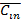# No-load test on a 3-phase induction motor was conducted at different supply voltages and a plot of input power versus voltage was drawn.This curve was extrapolated to intersect the Y-axis.This intersection point yields

1.  core loss

2.  stator copper loss

4.  friction and windage loss

4

friction and windage loss

Explanation :
No Explanation available for this question

# Bundled conductors are mainly used in high voltage overhead transmission lines to

1.  reduce transmission line losses

2.  increase mechanical strength of the line

3.  reduce corona

4.  reduce sag

4

reduce corona

Explanation :
No Explanation available for this question

# A power system consists of 300 buses out of which 20 buses are generator buses,25 buses are the ones with reactive power support and 15 buses are the ones with fixed shunt capacitors.All the other buses are load buses.It is proposed to perform a load flow analysis for the system using Newton-Raphson method.The size of the Newton-Raphson Jacobian matrix is

1.  553×553

2.  540×540

3.  555×555

4.  554×554

4

540×540

Explanation :
No Explanation available for this question

# Choose two appropriate auxillary components of a HVDC transmission system from the following P D.C.line inductor Q A.C.line inductor R Reactive power sources S Distance relays on D.C.line T Series capacitance of A.C. line

1.  P and Q

2.  P and R

3.  Q and S

4.  S and T

4

P and R

Explanation :
No Explanation available for this question

# A round rotor generator with internal voltage E1=2.0.p.u. and X=1.1.p.u is connected to a round rotor synchronous motor with internal voltage E2=1.3.p.u. and X=1.2.p.u.The reactance of the line connecting the generator to the motor is 0.5.p.u.when the generator supplies 0.5.p.u.power,the rotor angle difference between the machines will be

1.  57.42o

2.  1o

3.  32.58o

4.  122.58o

4

32.58o

Explanation :
No Explanation available for this question

# The interrupting time of a circuit breaker is the period between the instant of

1.  initiation of short circuit and the arc extinction on an opening operation

2.  energizing of the trip circuit and the arc extinction on an opening operation

3.  intiation of short circuit and the parting of primary arc contacts

4.  energizing of the trip circuit and the parting of primary arc contacts

4

energizing of the trip circuit and the arc extinction on an opening operation

Explanation :
No Explanation available for this question

# The variation of drain current with gate-to-source voltage (ID-VGS characteristics)of a MOSFET  is shown in the figure.The MOSFET is

1.  an n-channel depletion mode device

2.  an n-channel enhancement mode device

3.  a p-channel depletion mode device

4.  a p- channel enhancement mode device

4

a p- channel enhancement mode device

Explanation :
No Explanation available for this question

# In the circuit of the figure,assume that the transistor has hFE=99  and VBE=0.7V.The value of collector current IC of the transistor  is  approximately

1.  [3.3/3.3]mA

2.  [3.3/(3.3+3.3)]mA

3.  [3.3.33]mA

4.  [3.3/(33+3.3)]mA

4

[3.3/(3.3+3.3)]mA

Explanation :
No Explanation available for this question

# For the circuit of figure with an ideal operational amplifier,the maximum phase shift of the output Vout with reference to the input VIn is

1.  0o

2.  -90o

3.  +90o

4.  ±180o

4

±180o

Explanation :
No Explanation available for this question

# Figure shows a 4 to 1 MUX to be used to implement the sum of a 1-bit full adder with input bits P and Q and the carry input Cin.Which of the following combinations of inputs to I0,I1,I2 and I3 of the MUX will realize the sum S

1.  I0=I1=Cin;I2=I3=

2.   I0=I1= ;I2=I3= Cin

3.  I0=I3=Cin;I1=I2=

4.   I0=I3= ;I1=I2= Cin

4
I0=I3=Cin;I1=I2=